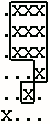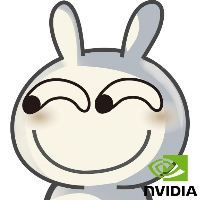## poj_1111Image Perimeters 原

Image Perimeters
 Time Limit: 1000MS Memory Limit: 10000K Total Submissions: 6891 Accepted: 4106

Description

Technicians in a pathology lab analyze digitized images of slides. Objects on a slide are selected for analysis by a mouse click on the object. The perimeter of the boundary of an object is one useful measure. Your task is to determine this perimeter for selected objects.

The digitized slides will be represented by a rectangular grid of periods, '.', indicating empty space, and the capital letter 'X', indicating part of an object. Simple examples are
``````XX   Grid 1       .XXX   Grid 2

XX                .XXX

.XXX

...X

..X.

X...``````

An X in a grid square indicates that the entire grid square, including its boundaries, lies in some object. The X in the center of the grid below is adjacent to the X in any of the 8 positions around it. The grid squares for any two adjacent X's overlap on an edge or corner, so they are connected.
``````XXX

XXX    Central X and adjacent X's

XXX``````

An object consists of the grid squares of all X's that can be linked to one another through a sequence of adjacent X's. In Grid 1, the whole grid is filled by one object. In Grid 2 there are two objects. One object contains only the lower left grid square. The remaining X's belong to the other object.

The technician will always click on an X, selecting the object containing that X. The coordinates of the click are recorded. Rows and columns are numbered starting from 1 in the upper left hand corner. The technician could select the object in Grid 1 by clicking on row 2 and column 2. The larger object in Grid 2 could be selected by clicking on row 2, column 3. The click could not be on row 4, column 3.One useful statistic is the perimeter of the object. Assume each X corresponds to a square one unit on each side. Hence the object in Grid 1 has perimeter 8 (2 on each of four sides). The perimeter for the larger object in Grid 2 is illustrated in the figure at the left. The length is 18.

Objects will not contain any totally enclosed holes, so the leftmost grid patterns shown below could NOT appear. The variations on the right could appear:
``````Impossible   Possible

XXXX         XXXX   XXXX   XXXX

X..X         XXXX   X...   X...

XX.X         XXXX   XX.X   XX.X

XXXX         XXXX   XXXX   XX.X

.....        .....  .....  .....

..X..        ..X..  ..X..  ..X..

.X.X.        .XXX.  .X...  .....

..X..        ..X..  ..X..  ..X..

.....        .....  .....  .....``````

Input

The input will contain one or more grids. Each grid is preceded by a line containing the number of rows and columns in the grid and the row and column of the mouse click. All numbers are in the range 1-20. The rows of the grid follow, starting on the next line, consisting of '.' and 'X' characters.

The end of the input is indicated by a line containing four zeros. The numbers on any one line are separated by blanks. The grid rows contain no blanks.

Output

For each grid in the input, the output contains a single line with the perimeter of the specified object.

Sample Input

``````2 2 2 2
XX
XX
6 4 2 3
.XXX
.XXX
.XXX
...X
..X.
X...
5 6 1 3
.XXXX.
X....X
..XX.X
.X...X
..XXX.
7 7 2 6
XXXXXXX
XX...XX
X..X..X
X..X...
X..X..X
X.....X
XXXXXXX
7 7 4 4
XXXXXXX
XX...XX
X..X..X
X..X...
X..X..X
X.....X
XXXXXXX
0 0 0 0``````

Sample Output

``````8
18
40
48
8``````
``````#include <iostream>
#include <cstring>
using namespace std;
#pragma warning(disable : 4996)
#define MAX 25

char map[MAX][MAX];
int visit[MAX][MAX];
int dir={{0,1},{1,1},{1,0},{1,-1},{0,-1},{-1,-1},{-1,0},{-1,1}};
int r, c, sum;

void dfs(int x, int y)
{
visit[x][y] = 1;
for(int i = 0; i < 8; i++)
{
int p = x + dir[i];
int q = y + dir[i];
if(p >= 1 && p <= r && q >= 1 && q <= c)
{
if(map[p][q] == 'X' && visit[p][q] == 0)
{
dfs(p, q);
}
else if(map[p][q]=='.' && (p == x || q == y))
{
sum++;
}
}

else if (p == x || q == y)
{
sum++;
}
}
}

int main()
{
freopen("in.txt", "r", stdin);
int i, j, x, y;
while(cin >> r >> c >> x >> y)
{
if(x + y + r + c == 0) break;
for(i = 1; i <= r; i++)
{
for(j = 1; j <= c; j++)
{
visit[i][j] = 0;
}
}
for(i=1; i <= r; i++)
{
for(j = 1; j <= c; j++)
{
cin >> map[i][j];
}
}
sum = 0;
dfs(x, y);
cout << sum << endl;
}
return 0;
}``````### N3verL4nd

js 不同页面间传递值并取值

2017/09/25
0
0

2016/04/02
156
1

ACM的竞赛性强,因此自己应该和自己的实际应用联系起来.适合自己的才是好的,有的人不适合搞算法,喜欢系统架构,因此不要看到别人什么就眼红,发挥自己的长处,这才是重要的. 第一阶段：练经典常用...

long0404
2015/06/24
0
0
POJ3723 《挑战程序设计竞赛》踩坑

2017/12/25
0
0
POJ的代码评审是如何实现的？

POJ上，提交一段代码，除了代码运行是否正确，还对程序的运行时间、空间都有限制，请问对程序运行的时空限制是如何做到的，通过编程控制（POJ支持的语言有c/c++/java/fortran/python/...）？...

J-will
2013/01/17
301
0

46分钟前
3
0
《JAVA核心知识》学习笔记（6. Spring 原理）-5

Shingfi
47分钟前
5
0
Excel导入数据库数据+Excel导入网页数据【实时追踪】

1.Excel导入数据库数据：数据选项卡------>导入数据 2.Excel导入网页数据【实时追踪】：

55分钟前
5
1

Airship

6
0

5
0